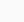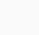# Problems And Solutions SEAMO PAPER B 2016

The Southeast Asia Mathematical Olympiad (SEAMO) is an international Math Olympiad competition that originated in Singapore and was founded by Mr. Terry Chew in 2016 in 8 Southeast Asian Countries. Since then, it is growing its popularity around the world. In 2019 it was recognized by 18 countries. In 2020 total number of participating countries increased to 22, including students from Indonesia, Brazil, China, Newzealand, and Taiwan students enrolled in SEAMO 2022-23.

Problem and Solution SEAMO 2016 paper B. Soal ini bersumber dari seamo-official.org

1. Find the missing number in the number pattern below.(A) 4
(B) 5
(C) 6
(D) 7
(E) None of the above

E

2. What is the sum of first 68 digits in

$$358421358421358421…$$

(A) 246
(B) 252
(C) 261
(D) 273
(E) 284

C

3. In a new operation

34 = 13,
59 = 24,
77 = 28,

Find the value of 138

(A) 45
(B) 46
(C) 47
(D) 48
(E) None of the above

C

4. The figure shown below is a 4 × 4 square grid.How many squares contain the dot?
(A) 8
(B) 10
(C) 12
(D) 14
(E) 16

B

5. Find the value ofin the following.(A) 23
(B) 29
(C) 37
(D) 39
(E) 41

E

6. Find the missing number.

$$[( ? + 4) ×8 ] ÷ 12 = 48$$

(A) 68
(B) 70
(C) 74
(D) 76
(E) None of the above

A

7. Find the value of S in the 3 × 3 magic square shown below.(A) 5
(B) 6
(C) 8
(D) 9
(E) 10

E

8. Find the value of

$$1 − 2 + 3 − 4 + 5 − 6 + ⋯+ 73 − 74 + 75$$

(A) 37
(B) 38
(C) 39
(D) 40
(E) None of the above

B

9. A bag contains 12 red, 10 white, 8 yellow, 3 blue and 2 black balls. Roy takes out the balls one by one without looking. At least how many balls must he take so that, for certain, he would have 4 balls of the same colour?(A) 15
(B) 19
(C) 20
(D) 25
(E) None of the above

E

10. Circles A, B and C have areas 380 cm², 400 cm² and 420 cm² respectively. The numbers represent overlapping areas in cm². Find the total area of the figure.(A) 780
(B) 820
(C) 960
(D) 1200
(E) None of the above

D

Pages ( 1 of 2 ): 1 2Next »